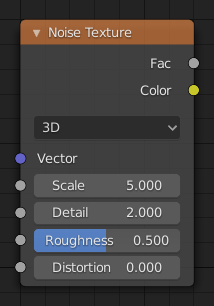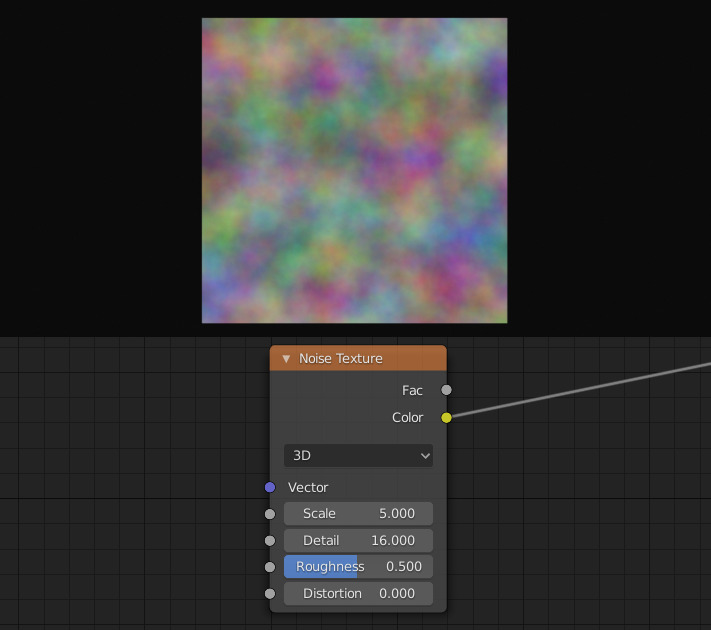# 噪波纹理着色器节点¶The Noise Texture node evaluates a fractal Perlin noise at the input texture coordinates.

## 输入选项¶

The inputs are dynamic, they become available if needed depending on the node properties.

Texture coordinate to evaluate the noise at; defaults to Generated texture coordinates if the socket is left unconnected.

W

Texture coordinate to evaluate the noise at.

Scale of the base noise octave.

Number of noise octaves. The fractional part of the input is multiplied by the magnitude of the highest octave. Higher number of octaves corresponds to a higher render time.

Roughness

Blend between a smoother noise pattern, and rougher with sharper peaks.

## 属性¶

Dimensions

The dimensions of the space to evaluate the noise in.

1D

Evaluate the noise in 1D space at the input W.

2D

Evaluate the noise in 2D space at the input Vector. The Z component is ignored.

3D

Evaluate the noise in 3D space at the input Vector.

4D

Evaluate the noise in 4D space at the input Vector and the input W as the fourth dimension.

Higher dimensions corresponds to higher render time, so lower dimensions should be used unless higher dimensions are necessary.

## 输出选项¶

Value of fractal noise.

Color with different fractal noise in each component.

## 举例¶